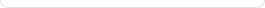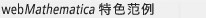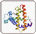Wolfram|Alpha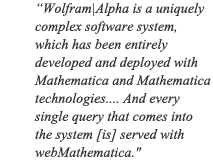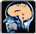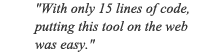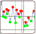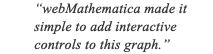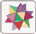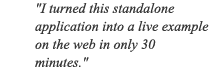Wolfram|Alpha

• webMathematica 每秒处理数千个查询，具有 Mathematica 全套计算和图形功能

• 采用与 Mathematica 内置的同一简明语法
• 使用 Mathematica 的全部图像处理函数

• 利用 Mathematica 高度个性化的符号图形功能，建立您需要的任何类型的互动图表。
• 运用高级统计与数据挖掘功能，检验数据。

• 自动生成并下载您计算结果的格式化报告。
• 访问 Mathematica 的内置可计算数据，包括几何数据。